JVxie's Blog 假装是个技术型博客（逃 2021-05-12T18:41:32.781Z http://jvxie.com/ Jeson Vendetta Xie Hexo [算法] 浅讲线段树和树状数组（一） http://jvxie.com/algorithm-segtree/ 2020-07-04T08:50:13.000Z 2021-05-12T18:41:32.781Z 写在前面

• C++语法基础
• 位运算基础
• 函数的递归和回溯思想
• 分治思想（如二分）
• 基础数据结构（主要为二叉树）

引入 & 建树

• 求某区间的和/最大值/最小值/乘积……
• 修改某个数字/某个区间段里的数字
• ……

——改编自百度百科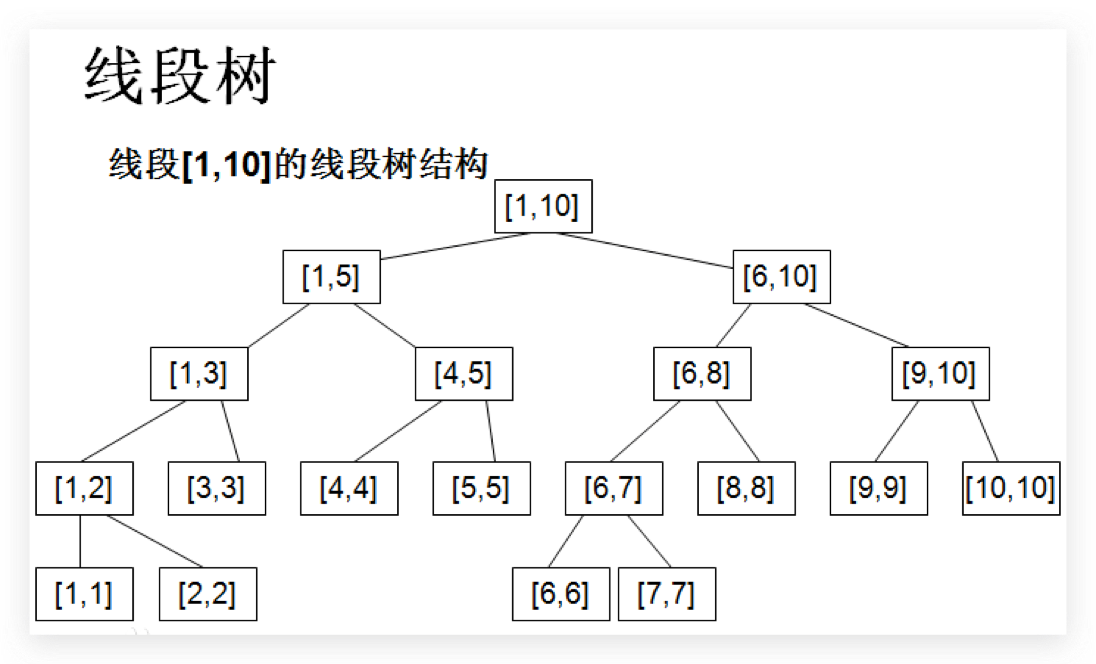• 线段树结构的节点数应以大于等于数据量的满二叉树计算（想想为什么？），所以实际申请的空间大致为最大区间长度的$4$倍。
• 若是合并儿子节点信息的操作比较复杂，可以独立成一个函数方便修改，这里只需要相加，所以直接写在建树里。

单点修改 & 单点取值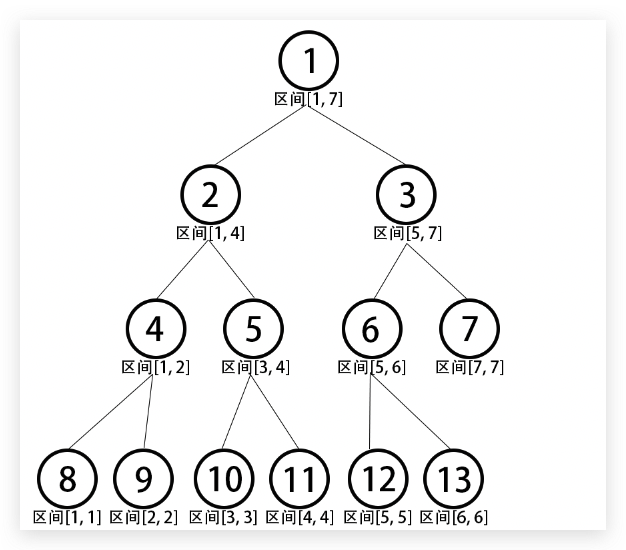*圆圈内数字为线段树节点的下标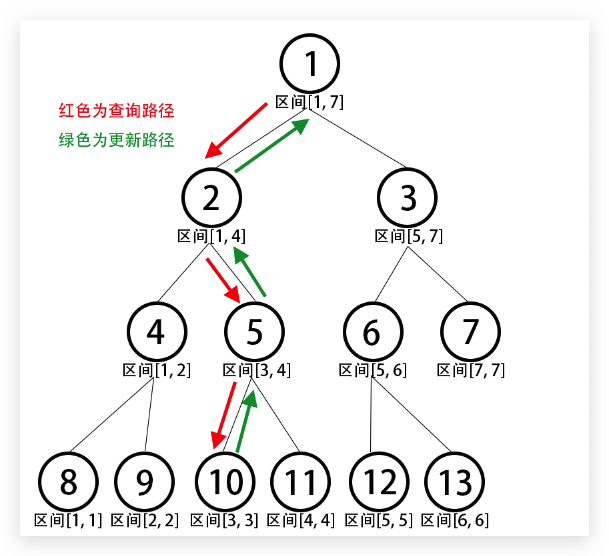区间查询 & 区间修改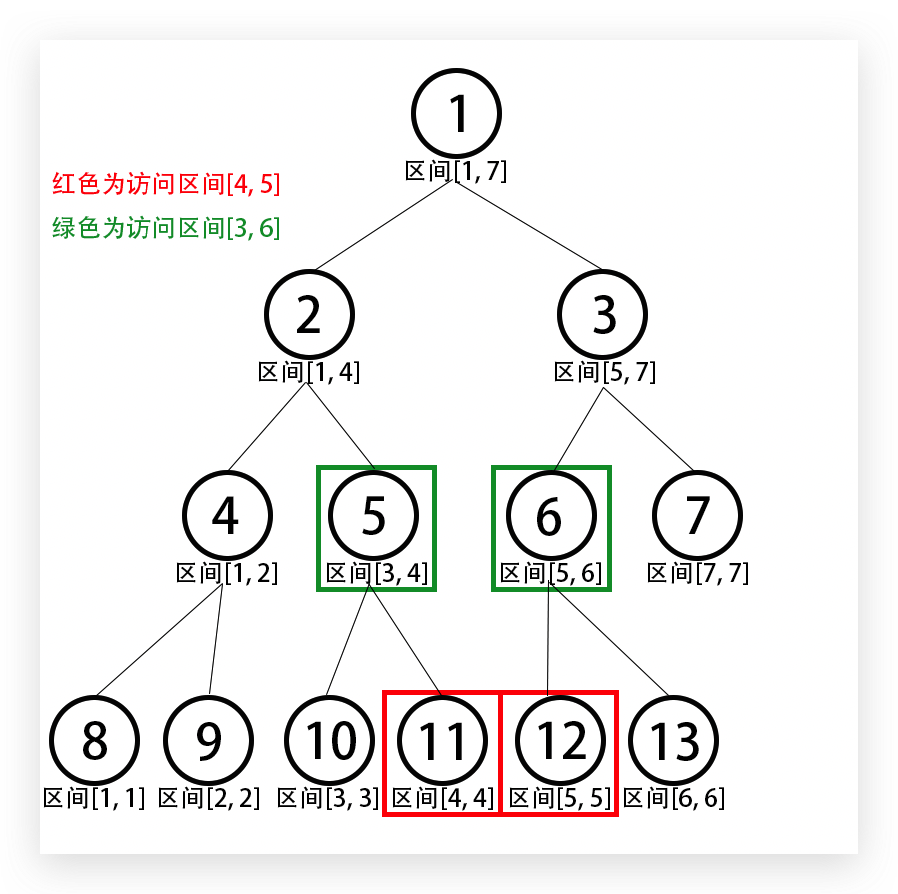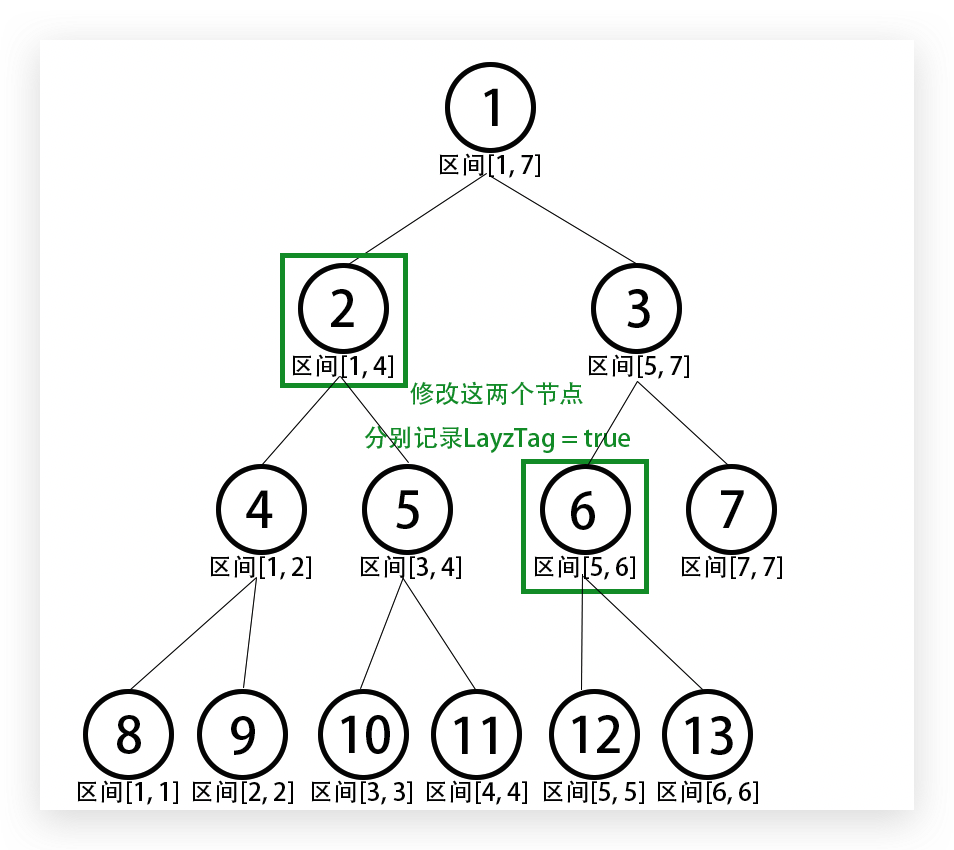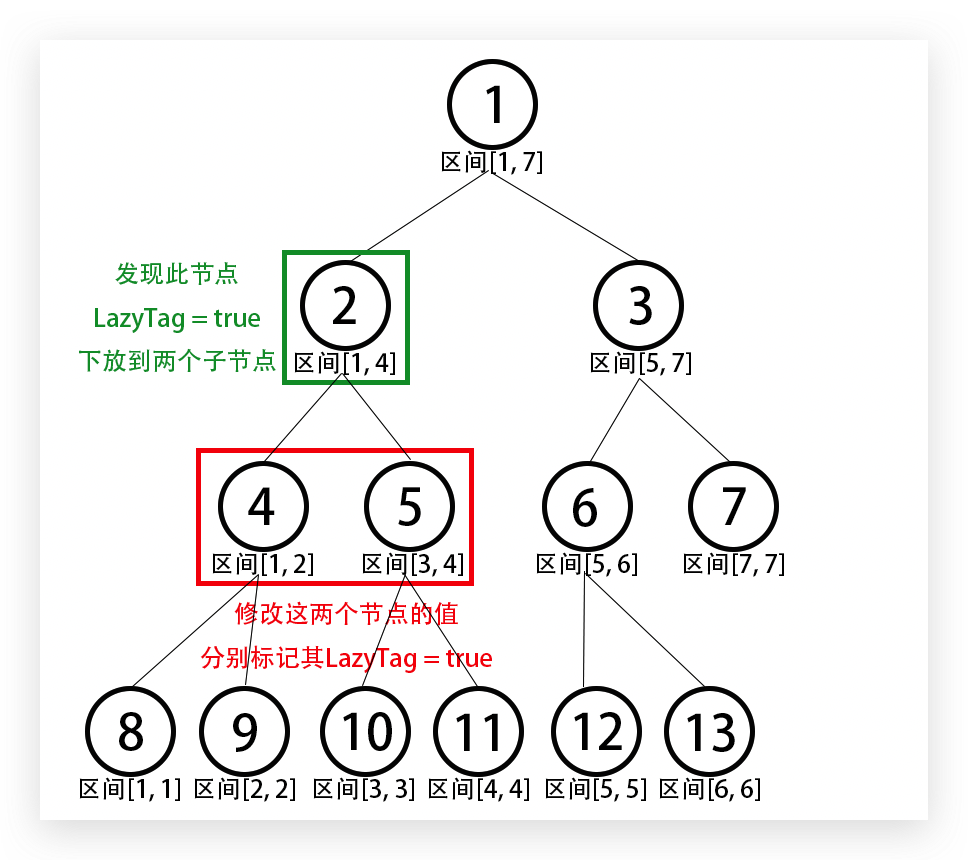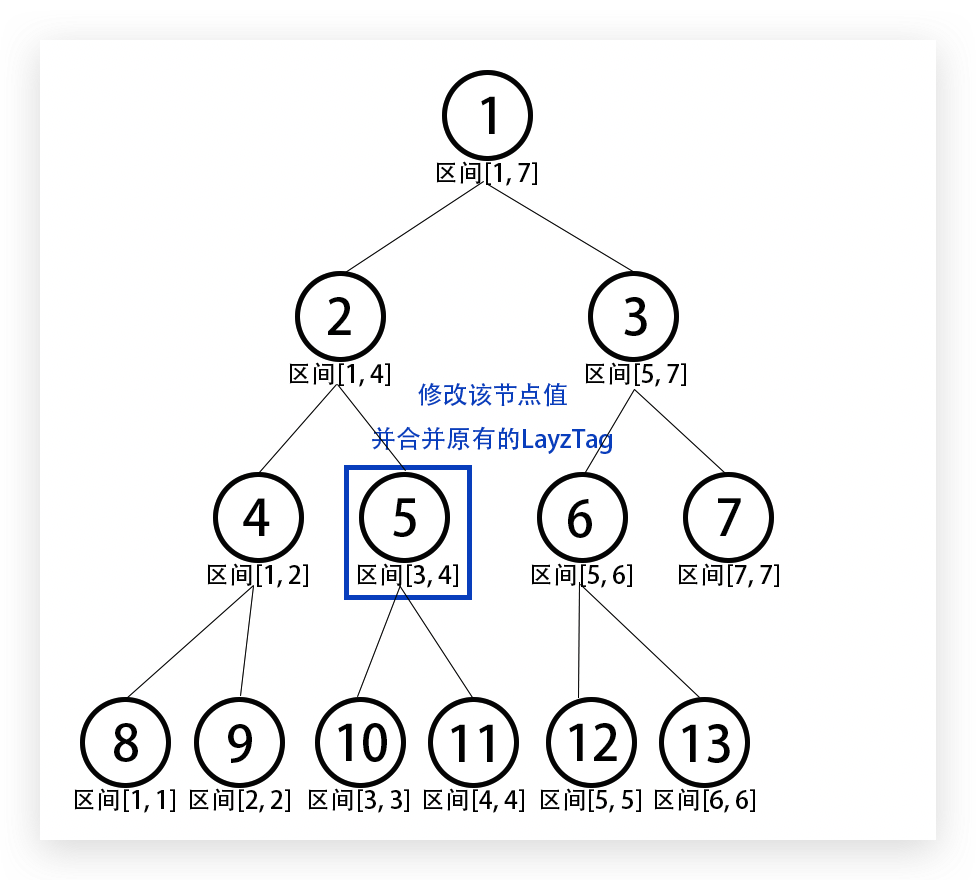线段树例题 & 小结

例题 - FZU 2297 Number theory

题目大意

• M $y_i$ 代表给$x$乘上$y_i$$1 \leq y_i \leq {10}^{9}$）。
• D $d_i$ 代表让$x$除以$y_{d_i}$。（原题目中这里有错误，操作符是D而不是N）

• 区间查询/修改
• 区间合并

权值线段树

]]>
<h1 id="写在前面"><a class="markdownIt-Anchor" href="#写在前面"></a> 写在前面</h1> <p>线段树是我最喜欢的数据结构之一了，几乎每一场比赛都能遇到相关的题目，它的强大是有目共睹的。</p> <p>这篇博客主要是讲讲它的原理
Python爬虫学习笔记（一）Scrapy框架实战 http://jvxie.com/spider-learning/ 2020-07-03T10:20:57.000Z 2020-07-03T18:09:20.231Z 写在前面

什么是Scrapy

Scrapy是一个为了爬取网站数据，提取结构性数据而编写的应用框架。 可以应用在包括数据挖掘，信息处理或存储历史数据等一系列的程序中。

Scrapy的框架如下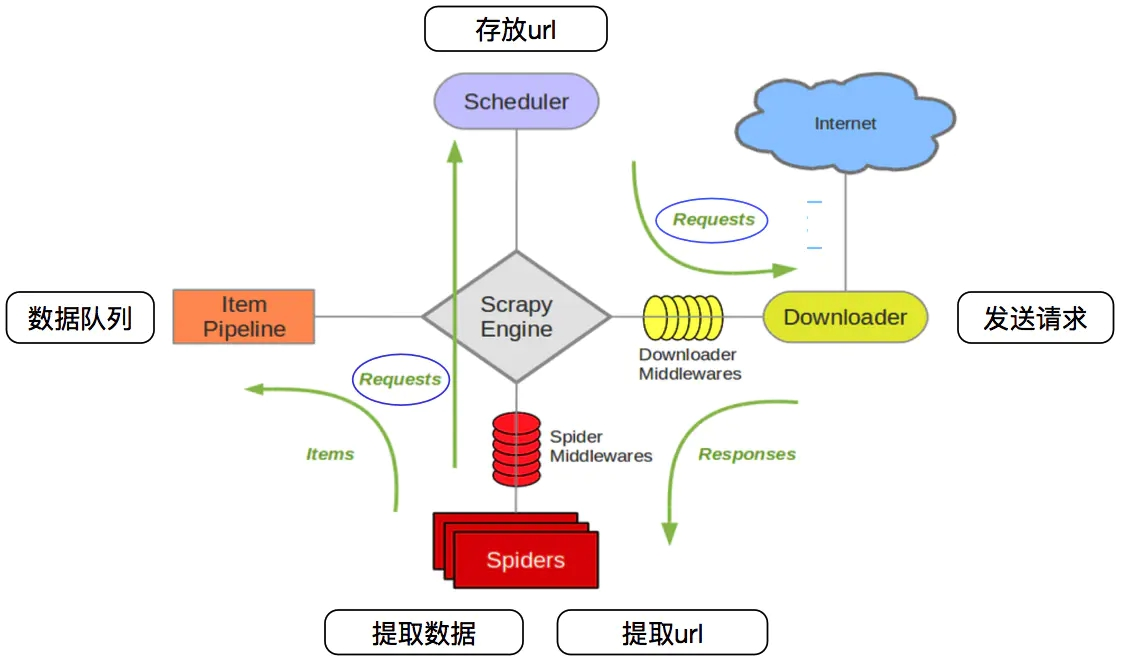• Scheduler(调度器): 它负责接受引擎发送过来的Request请求，并按照一定的方式进行整理排列，入队，当引擎需要时，交还给引擎。

• Spider（爬虫）：它负责处理所有Responses,从中分析提取数据，获取Item字段需要的数据，并将需要跟进的URL提交给引擎，再次进入Scheduler(调度器)，

• Item Pipeline(管道)：它负责处理Spider中获取到的Item，并进行进行后期处理（详细分析、过滤、存储等）的地方.

• Spider Middlewares（Spider中间件）：你可以理解为是一个可以自定扩展和操作引擎和Spider中间通信的功能组件（比如进入Spider的Responses;和从Spider出去的Requests）

开始使用

创建项目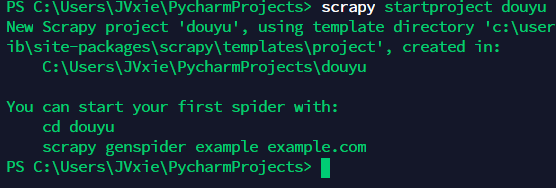• scrapy.cfg：项目的配置文件
• items.py：存放容器的文件
• middlewares.py：下载中间件和Spider中间件的定义和实现文件
• pipelines.py：管道的定义和实现文件，用于数据清洗、存储及验证
• settings.py：项目的设置文件
• spiders/：存放爬虫代码的目录

创建一个爬虫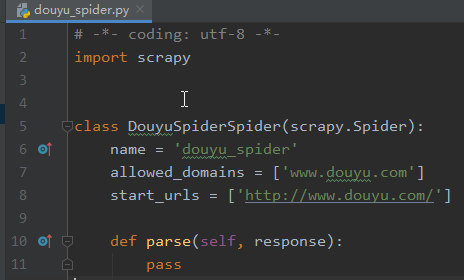• name： 用于区别Spider。 该名字必须是唯一的，您不可以为不同的Spider设定相同的名字。
• allowed_domains：包含了Sprider爬取的域名，防止爬取到其他域名
• start_urls ：包含了Spider在启动时进行爬取的url列表。 因此，第一个被获取到的页面将是其中之一， 后续的URL则从初始的URL获取到的数据中提取
• parse() ：将调用的方法，用于处理为每个请求下载的响应。响应参数是的实例 TextResponse它保存页面内容，并有进一步有用的方法来处理它

运行爬虫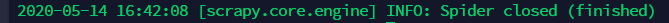实战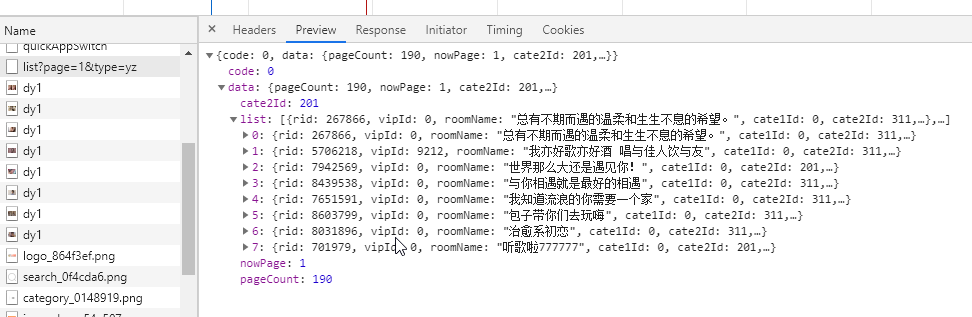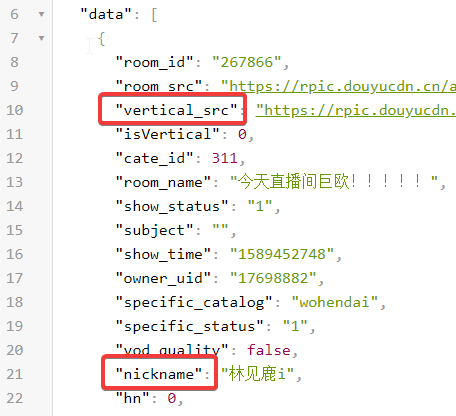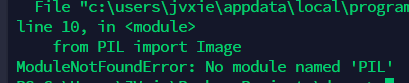]]>
<h1 id="写在前面"><a class="markdownIt-Anchor" href="#写在前面"></a> 写在前面</h1> <p>这篇博客来记录学习Python爬虫</p> <p>主要是为了应付数据可视化这门课程而写，虽然标题有（一），但可能不会有（二）了</p
Java Swing组件学习笔记 http://jvxie.com/Java-Swing-learning/ 2019-11-27T07:30:29.000Z 2020-06-28T16:23:57.381Z 写在前面

So，这篇博客讲的更多的会是计算器怎么做啦。

前期工作

什么是Swing组件？

Swing 是一个为Java设计的GUI工具包。

Swing是Java基础类的一部分。

Swing包括了图形用户界面（GUI）器件如：文本框，按钮，分隔窗格和表。

Swing提供许多比AWT更好的屏幕显示元素。它们用纯Java写成，所以同Java本身一样可以跨平台运行，这一点不像AWT。它们是JFC的一部分。它们支持可更换的面板和主题（各种操作系统默认的特有主题），然而不是真的使用原生平台提供的设备，而是仅仅在表面上模仿它们。这意味着你可以在任意平台上使用JAVA支持的任意面板。轻量级组件的缺点则是执行速度较慢，优点就是可以在所有平台上采用统一的行为。

它包含些什么？

JFrame – java的GUI程序的基本思路是以JFrame为基础，它是屏幕上window的对象，能够最大化、最小化、关闭。

JPanel – Java图形用户界面(GUI)工具包swing中的面板容器类，包含在javax.swing 包中，可以进行嵌套，功能是对窗体中具有相同逻辑功能的组件进行组合，是一种轻量级容器，可以加入到JFrame窗体中。

JLabel – JLabel 对象可以显示文本、图像或同时显示二者。可以通过设置垂直和水平对齐方式，指定标签显示区中标签内容在何处对齐。默认情况下，标签在其显示区内垂直居中对齐。默认情况下，只显示文本的标签是开始边对齐；而只显示图像的标签则水平居中对齐。

JTextField – 一个轻量级组件，它允许编辑单行文本。

JButton – JButton 类的实例。用于创建按钮。

……

• 顶层容器
• 菜单栏（特殊中间容器）
• 中间容器
• 基本组件

尝试编写

JFrame - 一切的开端

JFrame的常用默认构造方法有两个：

• JFrame() 创建默认无标题窗口

• JFrame(String) 创建带标题窗口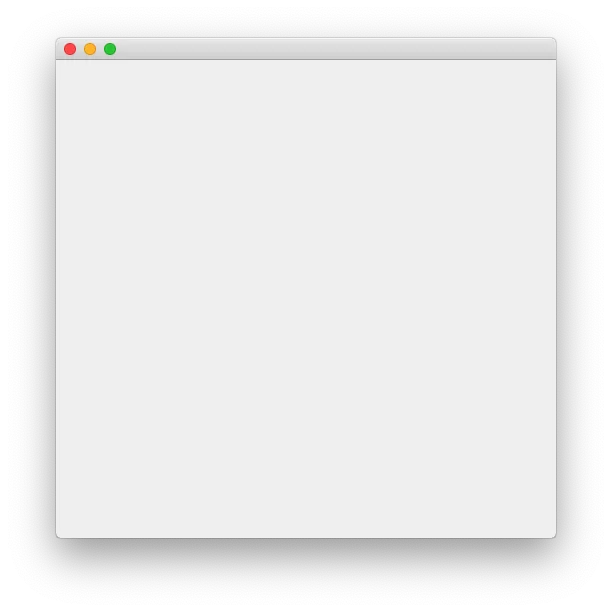setTitle(String)设置当前JFrame的标题
setResizable(boolean)设置当前JFrame可否调整大小
setDefaultCloseOperation(int)设置当前JFrame点击关闭按钮的动作
setVisible(boolean)设置当前JFrame是否可见
setSize(int, int)设置当前JFrame宽高
setLocationRelativeTo(Component)设置当前JFrame位置，null居中
Insets getInsets()返回当前JFrame边框元素

Q：为什么推荐使用继承的方式？

A：继承方式可以让我们更好地自定义（或者说DIY）JFrame，包括一些配置以及添加相关组件、监听事件等，对于Swing中含有的其他组件，也是推荐这样，当然前提是你需要DIY这个组件。

JPanel - 重点基础

JPanel的常用的构造方法有：

• JPanel() 创建默认使用流式布局的面板
• JPanel(LayoutManager) 创建指定布局的面板

JScrollPanel可滚动显示的面板
JSplitPane分隔面板
JTabbedPane选项卡面板
JLayeredPane层级面板

JButton - 初识布局

JButton常用的构造方法有：

• JButton() 创建无内容的按钮
• JButton(String) 创建含文本内容的按钮
• JButton(Icon) 创建有图标的按钮

setText(String)设置按钮显示的文本
setFont(Font)设置按钮显示的文本的字体
setForeground(Color)设置按钮文本的字体颜色
setIcon(Icon)设置按钮默认图标
setPressedIcon(Icon)设置按下时图标
setDisabledIcon(Icon)设置不可用时图标
doClick()模拟点击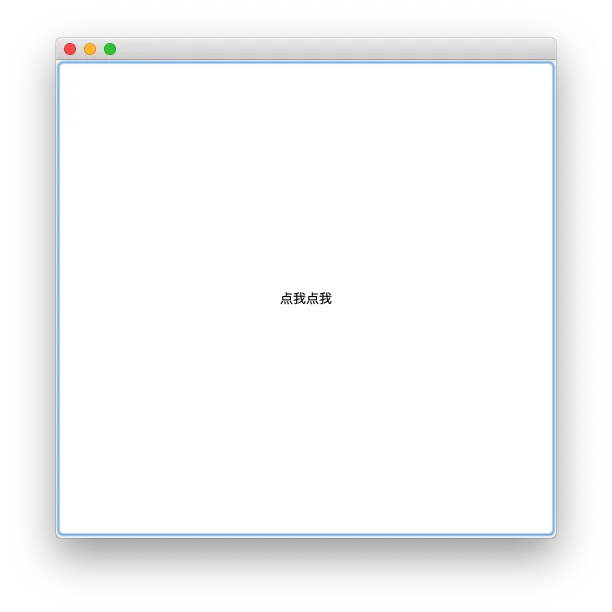FlowLayout流式布局，按组件加入顺序水平方向排列到当前组件，排满一行换下一行。
GirdLayout网格布局，将当前组件按指定行列数用网格分隔，每个网格放一个组件。
GridBagLayout网格袋布局，按网格将当前组件分割，每个组件可以占一个或多个网格，每个组件所占的网格大小可以用水平线和垂直线自定义。
BoxLayout箱式布局，将当前组件中的多个组件按水平或垂直方式排列。
GroupLayout分组布局，将当前组件按层次分组（串行或并行），分别确定组件组在水平和垂直方向的位置。
CardLayout卡片布局，将当前组件中的每个组件看作一张卡片，一次只显示一张，默认显示第一张。
BorderLayout边界布局，把当前组件按东西南北中五个方位分割，每个区域放一个组件。
SpringLayout弹性布局，通过定义组件四条边的坐标位置来实现布局。
null空（绝对）布局，对每个组件设置位置、大小放置到当前组件中。

• setPreferredSize(Dimension)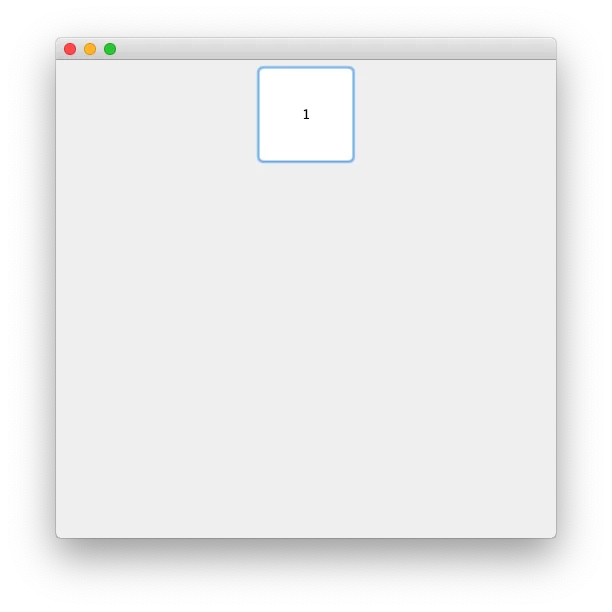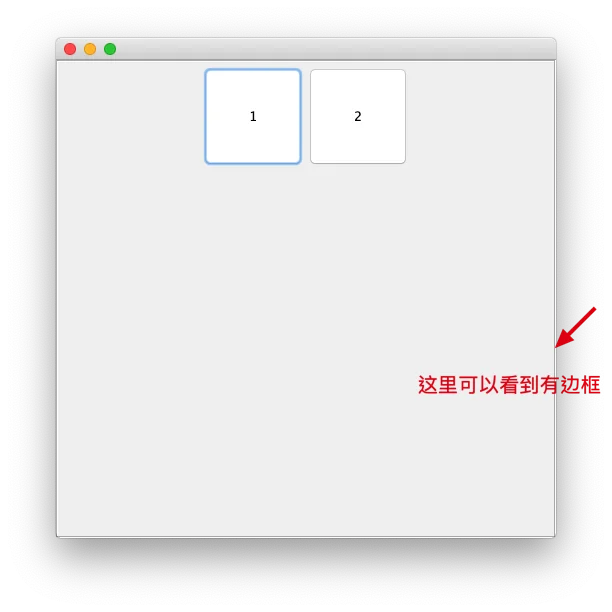]]>
<h1 id="写在前面"><a class="markdownIt-Anchor" href="#写在前面"></a> 写在前面</h1> <p>由于最近在做编译原理实验的作业——制作八、十、十六进制计算器，想要得高分需要做个界面。</p> <p>于是乎想到之前有学过的Java
[算法] 浅谈莫比乌斯反演 http://jvxie.com/algorithm-mobius/ 2019-09-05T09:40:42.000Z 2021-05-12T19:12:55.601Z 写在前面

前置知识

积性函数

定义

$gcd(x, y) = 1$$f(xy) = f(x)f(y)$，则称函数$f(x)$为积性函数。

性质

$f(x)$$g(x)$均为积性函数，则下列函数$h(x)$也为积性函数：

1. $h(x) = f(x^p)$
2. $h(x) = f^p(x)$
3. $h(x) = f(x)g(x)$
4. $h(x) = \displaystyle\sum_{d|x} {f(d)g(\frac{x}{d})}$

常见的积性函数

• 单位函数：$\varepsilon(n) = [n=1]$，也常写作$\varepsilon$
• 恒等函数：$id_k(n) = n^k$$id_1(n)$常写作$id(n)$
• 常数函数$\bold{1}(n) = 1$，常常直接用$\bold{1}$代替
• 除数函数：$\sigma_k(n) = \displaystyle\sum_{d|n}{d^k}$$\sigma_0(n)$通常记为$d(n)$，即常数函数，$\sigma_1(n)$通常记为$\sigma(n)$
• 欧拉函数：$\varphi(n) = \displaystyle\sum_{i=1}^{n} {[\gcd(i, n) = 1]}$
• 莫比乌斯函数： $\mu(d) = \begin{cases} 1, & {d = 0} \\ {(-1)} ^k, & {d = p_1 \cdot p_2 \dots p_k}, \forall p_i \not = p_j \\ 0, & {others} \end{cases}$

狄利克雷卷积

定义

$(f(n) * g(n))(n) = \displaystyle\sum_{d|n}{f(d)g(\frac{n}{d})}$

$(f(n) * g(n))(n)$常写作$f * g$

性质

1. 狄利克雷卷积满足交换律、结合律和分配律（前提是函数均满足加性）。

$f * g = g * f$

$(f * g) * h = f * (g * h)$

$f * (g + h) = f * g + f * h$

1. $\varepsilon$为狄利克雷卷积的幺元（单位元）。

$\forall f(x), x \in N_+ \implies f * \varepsilon = \varepsilon * f = f$

1. $f(x)$$g(x)$均为积性函数，则$f*g$也是积性函数，反之若$f*g$$g(x)$均为积性函数，则$f(x)$也是积性函数。

2. $g(x)$是完全积性函数，且$h(x) = f*g$，则$f(x) = h* (\mu \cdot g)$

一些例子

• $\mu * \bold{1} = \displaystyle\sum_{d|n}{\mu(d)} = \displaystyle\sum_{d|n}{\mu(\frac{n}{d})} = \bold{1} * \mu = \varepsilon$
• $\bold{1} * \bold{1} = \displaystyle\sum_{d|n}{1} = d(n)$
• $\mu * id = \displaystyle\sum_{d|n}{d \cdot \mu(\frac{n}{d})} = \varphi(n)$
• $\varphi * 1 = \displaystyle\sum_{d|n}{\varphi(\frac{n}{d})} = id(n)$

莫比乌斯函数

定义

$\mu(d) = \begin{cases} 1, & {d = 0} \\ {(-1)} ^k, & {d = p_1 \cdot p_2 \dots p_k}, \forall p_i \not = p_j \\ 0, & {others} \end{cases}$

性质

1. $\mu$是积性函数。
2. $\displaystyle\sum_{d|n}{\mu(d)} = \varepsilon$
3. $\displaystyle\sum_{d|\gcd(i, j)}{\mu(d)} = \varepsilon(\gcd(i, j)) = [\gcd(i, j) = 1]$
4. $\displaystyle\sum_{d|n} {\frac{\mu(d)}{d}} = {\frac{\varphi(n)}{n}}$

莫比乌斯反演

定义

$f(n)$$g(n)$为两个数论函数，如果

$f(n) = \displaystyle\sum_{d|n} {g(d)}$

$g(n) = \displaystyle\sum_{d|n} {\mu(d)f(\frac{n}{d})}$

$f(n) = \displaystyle\sum_{n|d} {g(d)} \rArr g(n) = \displaystyle\sum_{n|d} {\mu(\frac{d}{n})f(d)}$

证明

$\because f(n) = \displaystyle\sum_{d|n} {g(d)}$

$\therefore\displaystyle\sum_{d|n} {\mu(d)f(\frac{n}{d})} = \displaystyle\sum_{d|n} {\mu(d)} \displaystyle\sum_{k|\frac{n}{d}}{g(k)}$

$\displaystyle\sum_{d|n} {\mu(d)} \displaystyle\sum_{k|\frac{n}{d}}{g(k)} = \displaystyle\sum_{d|n}{g(d)} \displaystyle\sum_{k|\frac{n}{d}} {\mu(k)}$

$\because \displaystyle\sum_{d|n}{\mu(d)} = [n = 1] \rArr \displaystyle\sum_{k|\frac{n}{d}} {\mu(k)} = \bigg[\frac{n}{d} = 1\bigg]$

$\therefore \displaystyle\sum_{d|n}{g(d)} \displaystyle\sum_{k|\frac{n}{d}} {\mu(k)} = \displaystyle\sum_{d|n}{g(d) \cdot [n=d]} = g(n)$

$f = g * \bold{1} \rArr g = \mu * f$

$\mu * f = \mu * g * \bold{1} = g * (\mu * \bold{1}) = g * \varepsilon = g$

一些应用

简单例子：推导欧拉函数

$\sum_{i=1}^{n}{[\gcd(i, n)=1]},(1 \leq n \leq 10^7)$

$\sum_{i=1}^{n}{\sum_{d|gcd(i, n)}\mu(d)}$

$\begin{gathered} &\sum_{d=1}^{n}{\mu(d) \sum_{i=1}^{n}{[d|i]}} \\ = &\sum_{d=1}^{n}{\mu(d) \left\lfloor{\frac{n}{d}}\right\rfloor}\end{gathered}$

简单例子扩展

$\sum_{i=1}^{n}\sum_{j=1}^{m}{[\gcd(i, j)=k]},(1 \leq k \leq n \leq m \leq 10^5)$

$\sum_{i=1}^{\left\lfloor{\frac{n}{k}}\right\rfloor}\sum_{j=1}^{\left\lfloor{\frac{m}{k}}\right\rfloor}{[\gcd(i, j)=1]}$

$\begin{gathered} &\sum_{i=1}^{\left\lfloor{\frac{n}{k}}\right\rfloor}\sum_{j=1}^{\left\lfloor{\frac{m}{k}}\right\rfloor}{[\gcd(i, j)=1]} \\ = &\sum_{i=1}^{\left\lfloor{\frac{n}{k}}\right\rfloor}\sum_{j=1}^{\left\lfloor{\frac{m}{k}}\right\rfloor}{\sum_{d|gcd(i, j)}\mu(d)} \\ = &\sum_{d=1}^{\left\lfloor{\frac{n}{k}}\right\rfloor}{\mu(d)}\sum_{i=1}^{\left\lfloor{\frac{n}{k}}\right\rfloor}{[d|i]}\sum_{j=1}^{\left\lfloor{\frac{m}{k}}\right\rfloor}{[d|j]} \\ = &\sum_{d=1}^{\left\lfloor{\frac{n}{k}}\right\rfloor}{\mu(d)\left\lfloor{\frac{n}{kd}}\right\rfloor\left\lfloor{\frac{m}{kd}}\right\rfloor}\end{gathered}$

另一个简单例子：求最大公约数的和

$\sum_{i=1}^{n}{\gcd(i, n)}$

$\sum_{d|n}{\varphi(d)} = n \rArr \sum_{d|gcd(i, n)}{\varphi(d)} = \gcd(i, n)$

$\sum_{i=1}^{n}\sum_{d|gcd(i, n)}{\varphi(d)}$

$\sum_{d=1}^{n}{\varphi(d) \sum_{i=1}^{n}{[d|i]}} \rArr \sum_{d=1}^{n}{\varphi(d) \left\lfloor{\frac{n}{d}}\right\rfloor}$

$\sum_{i=1}^{n}{\sum_{j=1}^{m}\gcd(i, j)} = \sum_{d=1}^{n}{\varphi(d)\left\lfloor{\frac{n}{d}}\right\rfloor\left\lfloor{\frac{m}{d}}\right\rfloor}$

扩展：求最小公倍数的和

$\sum_{i=1}^{n}lcm(i, n) \rArr \sum_{i=1}^{n}{\frac{i \cdot n}{\gcd(i, n)}}$

$\begin{gathered} &\frac{1}{2} \cdot \left({\sum_{i=1}^{n-1}{\frac{i \cdot n}{\gcd(i, n)}} + \sum_{i=1}^{n-1}{\frac{(n-i) \cdot n}{\gcd(n-i, n)}}}\right) + n \\ = &\frac{1}{2} \cdot \sum_{i=1}^{n-1}{\frac{n^2}{\gcd(i, n)}} + n\end{gathered}$

$\frac{1}{2} \cdot \sum_{d

$\frac{n}{2} \cdot \sum_{d'>1,d'|n}^{}{d' \varphi(d')} + n$

$g(n) = \displaystyle\sum_{d'>1,d'|n}^{}{d' \varphi(d')}$，易知$g(n)$是积性函数，可以利用其性质进行线性筛。

$\begin{gathered} &\sum_{i=1}^{n}lcm(i, n) = \sum_{i=1}^{n}{\frac{i \cdot n}{\gcd(i, n)}} \\ = &n \cdot \sum_{d|n}^{} {\frac{1}{d}} \cdot {\sum_{i=1}^{n} [\gcd(i, n) = d] \cdot i} \\ = &n \cdot \sum_{d|n}^{} {\frac{1}{d}} \cdot {\sum_{i=1}^{\frac{n}{d}} [\gcd(i, \frac{n}{d}) = 1] \cdot i \cdot d} \\ = &n \cdot \sum_{d|n}^{} {\sum_{i=1}^{\frac{n}{d}} [\gcd(i, \frac{n}{d}) = 1] \cdot i}\end{gathered}$

$n \cdot \sum_{d|n}^{} \frac{\frac{n}{d} \cdot \varphi(\frac{n}{d})}{2}$

$n \cdot \sum_{d'|n}^{} \frac{d' \varphi(d')}{2}$

$n \cdot \sum_{d'>1, d'|n}^{} \frac{d' \varphi(d')}{2} + n$

$\frac{n}{2} \cdot \left(\sum_{d'|n}^{}{d' \varphi(d')} + 1\right)$

再扩展：二维最小公倍数求和

$\sum_{i=1}^{n}{\sum_{j=1}^{m}lcm(i, j)} \rArr \sum_{i=1}^{n}{\sum_{j=1}^{m}{\frac{i \cdot j}{\gcd(i, j)}}}$

$\begin{gathered} = &\sum_{i=1}^{n}{\sum_{j=1}^{m}{\sum_{d=1}^{n}{\frac{i \cdot j}{d} \cdot [\gcd(i, j) = d]}}} \\ = &\sum_{d=1}^{n}{\sum_{i=1}^{\lfloor\frac{n}{d}\rfloor}{\sum_{j=1}^{\lfloor\frac{m}{d}\rfloor}{\frac{i \cdot j \cdot d^2}{d} \cdot [\gcd(i, j) = 1]}}} \\ = &\sum_{d=1}^{n}{d \sum_{i=1}^{\lfloor\frac{n}{d}\rfloor}{\sum_{j=1}^{\lfloor\frac{m}{d}\rfloor}{i \cdot j \cdot [\gcd(i, j) = 1]}}} \\ = &\sum_{d=1}^{n}{d \sum_{i=1}^{\lfloor\frac{n}{d}\rfloor}{\sum_{j=1}^{\lfloor\frac{m}{d}\rfloor}{i \cdot j \cdot \sum_{k|\gcd(i,j)}^{}{\mu(k)}}}} \\ = &\sum_{d=1}^{n}{d \cdot \sum_{k=1}^{\lfloor\frac{n}{d}\rfloor}{\mu(k)} \cdot k^2 \cdot \sum_{i=1}^{\lfloor\frac{n}{dk}\rfloor}{\sum_{j=1}^{\lfloor\frac{m}{dk}\rfloor}{i \cdot j}}} \\\end{gathered}$

]]>
<h1 id="写在前面"><a class="markdownIt-Anchor" href="#写在前面"></a> 写在前面</h1> <p>这篇博客用来记录一下我学习莫比乌斯反演的过程，以及我是如何理解它的。</p> <p>期间参考了大量其他博客，这里列出表示感谢：</p
[题解] 头脑风暴专题 http://jvxie.com/solution-brainstorming/ 2019-08-28T06:58:04.000Z 2021-05-12T19:14:28.757Z 写在前面

LightOJ 1095 math

题解思路

$Ans = C_m^k * \displaystyle\sum_{i=0}^k {(C_{n-m}^i * d_{n-k-i})}$

NOIP2017 Day1 T1 小凯的疑惑

题解思路

$\therefore Ans = (b-1) * a - b = a * b - a - b$

BZOJ 3884 上帝与集合的正确用法

题目大意

$\underbrace{2^{2^{2^{\sdot^{\sdot^{\sdot^{2}}}}}}}_\infty \mod p$

CodeForces 977D Divide by three, multiply by two

题目大意

1. $x$除以$3$，前提是$x \mod 3 = 0$
2. $x$$2$

题解思路

$x_{i+1} = 3^{j_{i+1}} \cdot 2^{k_{i+1}} \cdot p$必然满足$j_{i+1} \leq j_i$$k_{i+1} \geq k_i$，那么我们只要按照这样的规则去对原有序列进行排序，就能找到一个满足题目要求的序列。

计蒜客 41229 super_log

题目大意

$\log_a^*(x) = \begin{cases} -1, &{x < 1} \\ 1 + \log_a^*(\log_a{x}), &{x \geq 1} \end{cases}$

题解思路

$f(a, n, p) = {}^n\!a \mod p$，则根据欧拉降幂公式得

$f(a, n, p) = \begin{cases} a^{f(a, n-1, \varphi(p))}, & {\gcd(a, p) = 1} \\ {}^n\!a, & {\gcd(a, p) \not = 1, ({}^{n-1}\!a) < p} \\ a^{f(a, n-1, \varphi(p)) + \varphi(p)}, & {\gcd(a, p) \not = 1,({}^{n-1}\!a) \geq p}\end{cases}$

快跑啊，JV又来抓人了！

题解思路

1. 由于$gcd(a, b) = gcd(b, a \mod b)$，那么我们便可以先求$\displaystyle\prod_{i=1}^{n }a_i \mod x$ ，这里可以利用__int128（如果编译器支持的话），或是写个高精度乘法和高精度取模求。

2. 对于$gcd(a \cdot b, x)$，易知其因子里必然含有$gcd(a, x)$，那么让$x$除以$gcd(a, x)$后再与$b$取最大公约数，再乘上$gcd(a, x)$就得到了$gcd(a \cdot b, x)$，即我们可以拆分成$gcd(a, x) \cdot gcd(b, x \div gcd(a, x))$，推广到$n$个数的话就是$gcd(\displaystyle\prod_{i=1}^{n }a_i, x) = gcd(\displaystyle\prod_{i=1}^{n-1 }a_i, x) \cdot gcd(a_n, x \div gcd(\displaystyle\prod_{i=1}^{n-1 }a_i, x))$，这样我们就得到了一个递推式，便可以在$O(n)$的时间复杂度内求出$x \div gcd(\displaystyle\prod_{i=1}^{n }a_i, x)$

AC代码

• 方法1：
• 方法2：
]]>
<h1 id="写在前面"><a class="markdownIt-Anchor" href="#写在前面"></a> 写在前面</h1> <p>这篇博客记录一下这些天来做（和看到）的思维题，昨天是越做越有趣，越做越起劲（其实是奶茶喝多了睡不着），直接导致了我又一不小心想出了一
[题解] HDU6703 array http://jvxie.com/solution-HDU6703-array/ 2019-08-26T11:45:13.000Z 2020-06-28T16:23:57.383Z 题目大意

1. $(1, pos)$：将$a_{pos}$修改为$a_{pos} + 10,000,000$
2. $(2, r, k)$：询问一个${a_1, a_2, ……, a_r}$之中未出现的最小正整数$Ans$且满足$Ans \geq k$

• 每组测试数据的第一个操作前定义一个$LastAns$初始化为$0$
• 若给出两个数$(1, t1)$，代表这次操作是操作$1$，最终的$pos = t1 \bigoplus LastAns$